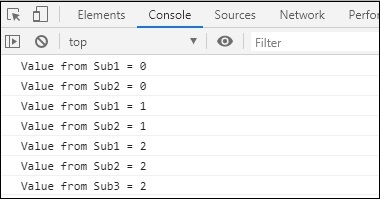# RxJS - Multicasting Operator share

It is an alias for multicast() operator with the only difference is that you don't have to called connect () method manually to start the subscription.

## Syntax

```share()
```

## Example

```import { interval} from 'rxjs';
import { take, share} from 'rxjs/operators';

let observer = interval(1000).pipe(take(3), share());
const subscribe_one = observer.subscribe(
x => console.log("Value from Sub1 = "+x)
);
const subscribe_two = observer.subscribe(
x => console.log("Value from Sub2 = "+x)
);
setTimeout(() => {
const subscribe_three = observer.subscribe(
x => console.log("Value from Sub3 = "+x)
);
}, 2000);
```

## Output